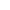Maths | AP Board | Grade 12 | 2016
Q. Find the area of the triangle formed by joining the mid points of the sides of the triangle, whose vertices are (0, 1);(2, 1) and (0, 3). Find the ratio of this area to the area of the given triangle
View SolutionQ. Find the number of terms in the following A.P. 7, 13, 19, ...., 205
View SolutionQ. If 52160=132n×5m, then m+n= ______
View SolutionQ. Find the area of the triangle, whose vertices are (2, 0), (1, 2), (1, 6). What do you observe?
View SolutionQ. Draw the Venn diagrams of the sets (AB), (BA)
View SolutionQ. A chord of a circle of radius 10 cm subtends a right angle at the centre. Find the area of the corresponding (i) Minor segment, (ii) Major segment. (use π=3.14)
View SolutionQ. Find a quadratic polynomial, if the zeroes of it are 2 and 1 respectively.
View SolutionQ. Find the roots of the equation 2x2+x6=0 by factorisation.
View SolutionQ. Solve the pair of equations by Elimination method.
2x+y5=0, 3x2y4=0
View SolutionQ. Solve the equations
10x+y+2xy=4, 15x+y5xy=2
View SolutionQ.

The distribution below gives the weights of 30 students of a class. Find the median weight of the students.

Weight(in Kgs)4045455050555560606565707075Number of students2386632

View SolutionQ. Expand log343125
View SolutionQ. Solve the following equations graphically.
3xy=7,
2x+3y=1
View SolutionQ. Find the common ratio of G.P.
2, 22, 4, ....
View SolutionQ. 10 students of class X took part in a mathematics quiz. If the number of girls is four more than the number of boys, then find the number of boys and the number of girls who took part in the quiz
View SolutionQ. Decimal form of 3623×53 value is ________
View SolutionQ. If p(x)=x25x6; find the value of p(3)
View SolutionQ. L.C.M. and H.C.F. of two numbers are 108 and 9 respectively. If one of the two numbers is 54, then the other number is _______
View SolutionQ. Find the mid point of the line segment joining the points (2, 7) and (12, 7)
View SolutionQ. If A={1, 2, 3, 4}, B={1, 2, 3, 5, 6}, then find
(i) AB, (ii) BA, (iii) AB, (iv) BA.
What do you observe?
View SolutionQ. If the sum of the first 7 terms of an A.P. is 49 and that of 17 terms is 289; find the sum of the first n terms
View SolutionQ. If log2x=3, then x= ______
View SolutionQ. Show that (53) is irrational.
View SolutionQ. Solve the quadratic equation 2x2+x4=0 by completing the square
View SolutionQ. Find the zeroes of the polynomial p(x)=x24x+3 and verify the relationship between zeroes and coefficients
View SolutionQ. Write the following set {x:x=2n+1 and nN} in roster form
View SolutionQ. List all the subsets of the following set B={p, q}
View SolutionQ. Find the value of log813
View SolutionQ. Draw the graph of p(x)=x2+3x4 and find zeroes.
Verify the zeroes of the polynomials
View SolutionQ. Find the coordinates of the point, which divides the join of (1, 7) and (4, 3) in the ratio 2:3
View Solution